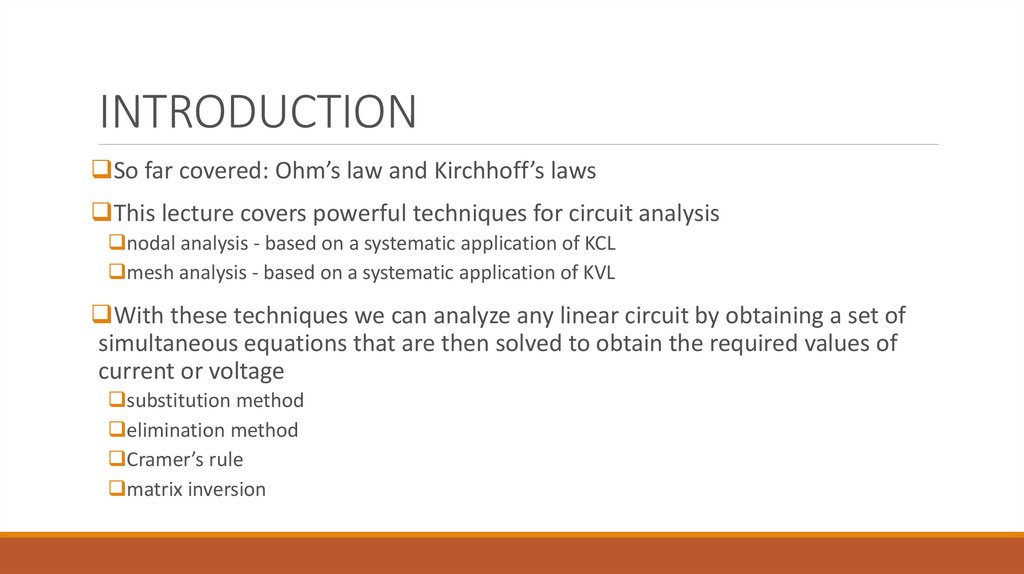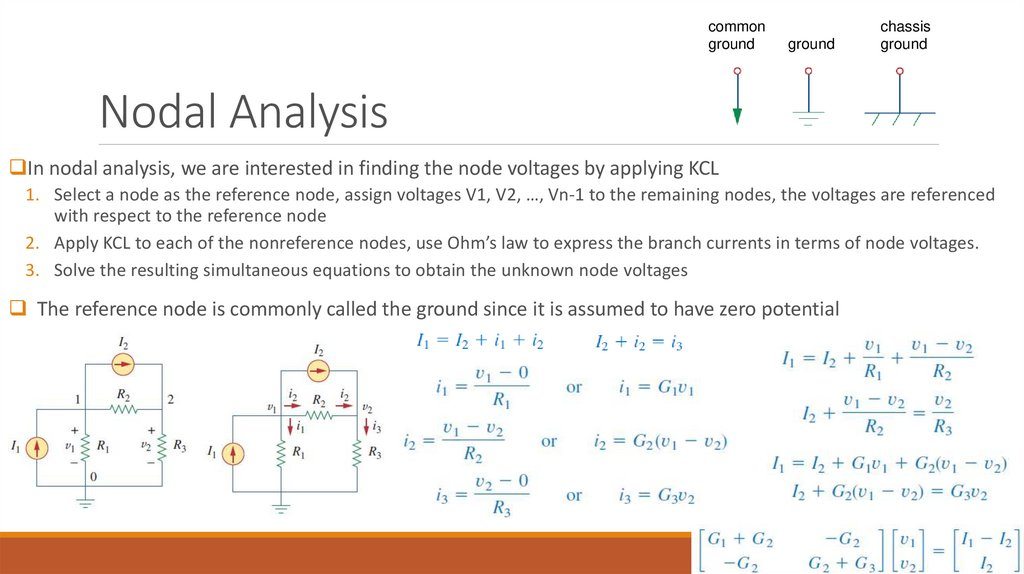Fundamentals of Electrical Engineering

1. Fundamentals of Electrical Engineering

COMPILED BY RASSIM SULIYEV

2. INTRODUCTION

So far covered: Ohm’s law and Kirchhoff’s laws
This lecture covers powerful techniques for circuit analysis
nodal analysis - based on a systematic application of KCL
mesh analysis - based on a systematic application of KVL
With these techniques we can analyze any linear circuit by obtaining a set of
simultaneous equations that are then solved to obtain the required values of
current or voltage
substitution method
elimination method
Cramer’s rule
matrix inversion

3. Nodal Analysis

common
ground
ground
chassis
ground
Nodal Analysis
In nodal analysis, we are interested in finding the node voltages by applying KCL
1. Select a node as the reference node, assign voltages V1, V2, …, Vn-1 to the remaining nodes, the voltages are referenced
with respect to the reference node
2. Apply KCL to each of the nonreference nodes, use Ohm’s law to express the branch currents in terms of node voltages.
3. Solve the resulting simultaneous equations to obtain the unknown node voltages
The reference node is commonly called the ground since it is assumed to have zero potential

4. Example

Calculate the node voltages in the circuit shown
The fact that i2 is negative shows that the current flows in
the direction opposite to the one assumed

5. Problems

Determine the voltages at the nodes

6. Problems

Determine the voltages at the nodes

7. Nodal Analysis with Voltage Sources

CASE 1: If a voltage source is connected between the reference node and a nonreference node, we simply set
the voltage at the nonreference node equal to the voltage of the voltage source
CASE 2: If the voltage source (dependent or independent) is
connected between two nonreference nodes, the two nonreference
nodes form a generalized node or supernode; we apply both KCL and
KVL to determine the node voltages
A supernode is formed by enclosing a (dependent or independent)
voltage source connected between two nonreference nodes and any
elements connected in parallel with it.

8. Example

For the circuit shown find the node voltages.

9. Problems

Find v and i in the circuit

10. Problems

Find v and i in the circuit
V1 = 14V
I1
V3
V2
I2
I3
I4
V1 = 14 V
42 = 7*V2 + 48 + 8*V2
6 = V3 - V2
15*V2 = -6
V3 = 6 + V2
V2 = -6/15 = -0.4 V
I1 = I2 + I3 + I4
V3 = 6 + (-0.4) = 5.6 V
(14 – V2)/4 = V2/3 + V3/2 + V3/6
I = V3/2 = 5.6 / 2 = 2.8 A
42 – 3*V2 = 4*V2 + 6*V3 + 2*V3
42 = 7*V2 + 8*V3
42 = 7*V2 + 8*(6 + V2)

11. Mesh Analysis (Planar / Nonplanar)

mesh analysis applies KVL to find mesh currents
Mesh analysis is not quite general because it is only applicable to a circuit that is planar
planar circuit is one that can be drawn in a plane with no branches crossing one another
Nonplanar circuits can be handled using nodal analysis
Planar circuit
Nonplanar circuit

12. Mesh Analysis

A mesh (independent loop) is a loop which does not contain any other loops within it
1.
Assign mesh currents I1, I2, …, In to the n meshes
2.
Apply KVL to each of the n meshes. Use Ohm’s law to express the voltages in terms of the mesh currents
3.
Solve the resulting n simultaneous equations to get the mesh currents.

13. Example

For the circuit, find the branch currents using mesh analysis

14. Problem

Use mesh analysis to find the current I0 in the circuit

15. Problem

Use mesh analysis to find the current I0 in the circuit

17. Mesh Analysis with Current Sources

the presence of the current sources reduces the number of equations
CASE 1: When a current source exists only in one mesh we set mesh
current equal to algebraic sum of current sources in that mesh
CASE 2: When a current source exists between two meshes we create
a supermesh by excluding the current source and any elements
connected in series with it, and apply KVL around supermesh

18. Example

For the circuit, find i1 to i4 using mesh analysis
i3 = i2 + 3*i4
i4 = 0.8*i3 - 1
i3 = i2 + 3*(0.8*i3 - 1)
i3 = i2 + 2.4*i3 – 3
i3 = (3 – i2)/1.4
I3 = (– i1 – 2)/1.4
I4 = 0.8*(– i1 – 2)/1.4 - 1
i1 + 3*(i1 + 5) + 6*(– i1 – 2)/1.4 – 4*(0.8*(– i1 – 2)/1.4 – 1) = 0
i1 + 3*i1 + 15 – 4.3*i1 – 8.6 + 4 + 2.3*i1 + 4.6 = 0
2*i1 +15 = 0
i1 = -7.5A
i2 = -2.5A
i3 = 3.93A
i4 = 2.143A

19. Nodal and Mesh Analyses by Inspection

Voltage of the selected node multiplied by sum
of conductances connected to that node minus
all neighboring nodes multiplied with
conductance that makes that node neighbor to
the selected one equals to algebraic sum of
current sources at the selected node
(R1+R3)*i1 – R3*i2 = v1
(R2+R3)*i2 – R3*i1 = -v2
(G1+G2)*v1 – G2*v2 = I1 – I2
(G2+G3)*v2 – G2*v1 = I2
Current of the selected mesh multiplied by sum of resistanses
around that mesh minus all neighboring meshes multiplied
with resistance that makes that mesh neighbor to the selected
one equals to algebraic sum of voltage sources around the
selected mesh

20. Nodal Versus Mesh Analysis

first factor is the nature of the particular network
Networks that contain many series-connected elements, voltage sources, or supermeshes are more
suitable for mesh analysis
whereas networks with parallel-connected elements, current sources, or supernodes are more suitable
for nodal analysis
circuit with fewer nodes than meshes is better analyzed using nodal analysis
circuit with fewer meshes than nodes is better analyzed using mesh analysis
second factor is the information required
If node voltages are required, it may be better to apply nodal analysis
If branch or mesh currents are required, it may be better to use mesh analysis

21. Home Work

From book Fundamentals of Electric Circuits (FIFTH EDITION) by Charles K. Alexander and